# 2nd PUC Basic Maths Previous Year Question Paper March 2018

Students can Download 2nd PUC Basic Maths Previous Year Question Paper March 2018, Karnataka 2nd PUC Maths Model Question Papers with Answers helps you to revise the complete Karnataka State Board Syllabus and score more marks in your examinations.

## Karnataka 2nd PUC Basic Maths Previous Year Question Paper March 2018

Time: 3 Hours 15 Min.
Max.Marks: 100

Instructions:

• The question paper has 5 parts A, B, C, D and E. Answer all the pats.
• Part-A carries 10 marks\, Parts – B carries 20 marks, Part – C carries 3 marks, Part – D carries 30 marks and Pat- E carries 1 marks.
• Write the question number properly as indicated in the question paper.

Part – A

Answer all the ten questions: (10 × 1 = 10)

Question 1.
If A = $$\left[ \begin{matrix} 2 & 4 \\ 3 & -1 \\ 4 & 0 \end{matrix} \right]$$ Show that (A’) = AQuestion 2.
How many different 4 digit number can be formed using the digits 1,2,4,5, 7, 8, 9. No digit being repeated.
Required arrangement = 7P4 =840

Question 3.
Symbolise the proposition.
2 + 5 = 6 or all integers are rationals.
P : 2 + 5 = 69: All integers are rationals Symbol: p ∨ q.Question 4.
Find the duplicate ratio of 5 : 3.
Duplicate ratio of 5 : 3 = 52 : 32 = 25 : 9

Question 5.
What rate of interest is obtained by investing in 9% stock at 180?
Yield = $$\frac{9}{180} \times 100$$ = 5%

Question 6.
If sin A = $$\frac { 3 }{ 5 }$$, find the 2A.
Sin 2A = 2 sin ACos A = $$2 \cdot \frac{3}{5} \cdot \frac{4}{5}=\frac{24}{25}$$

Question 7.
Find the equation of directrix for a given parabola x2 = 6y
4a = 6 a = $$\frac{3}{2}$$ Equation of directrix y = $$\frac{-3}{2}$$

Question 8.
Evaluate: $$\lim _{x \rightarrow 0} \frac{\sin a x}{b x}$$
$$\lim _{x \rightarrow 0}=\frac{\sin a x}{a x} \cdot \frac{a}{b}=\frac{a}{b}$$

Question 9.
If y = 5ax – log x= 3√x find any $$\frac{\mathrm{d} y}{\mathrm{d} x}$$
$$\frac{d y}{d x}=5 a^{x} \cdot \log a-\frac{1}{x}=\frac{3}{2 \sqrt{x}}$$

Question 10.
Evaluate: $$\int \frac{1}{5 e^{-x}} d x$$
$$\frac{1}{5} \int e^{x} d x=\frac{1}{5} e^{x}+c$$

Part – B

Answer any ten questions: (10 × 2 = 20 )

Question 11.
Solve by Cramer’s rule 3x + 4y = 7 and 7x – y = 6.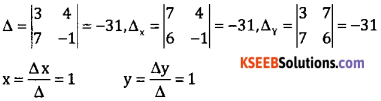Question 12.
Find n, if nP3 = 210.
nP3 = 210 n(n – 1) (n – 2) = 7 (7 – 1) (7 – 2)
∴ n = 7Question 13.
Two dice are thrown at once. What is the probability of getting face upwards with “sum equal to 4 or 5”.
A: sum equal to 4 = {(1, 3)(2, 2)(3, 1)}
B: sum equal to 5 = {(1,4)(2,3)(3,2)(4,1)}
P(A ∪ B) = P(A) + P(B) = $$\frac{3+4}{36}=\frac{7}{36}$$

Question 14.
If the truth valued of propositions p,q,r ar T,T,F respectively. Then find the truth values of the compound propositions (p ∨ r) ∧ q.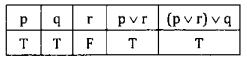Question 15.
Monthly income of A and B are in the ratio 2:3 and their monthly expenditure are in the ratio 3:5. If each saves ₹ 100 per month. Find the monthly incomes of A and B.
Let the monthly incomes be 2x and 3x respectively since their savings are ₹ 100 their expenditure would be
(2x – 100) and (3x – 100) Given $$\frac{2 x-100}{3 x-100}=\frac{3}{5}$$ ⇒ x = ₹100
∴ Monthly incomes of A = ₹ 400
Monthly income of B = ₹ 600

Question 16.
A bill drawn for 3 month was legally due on 06.07.2018. Find the date of drawing of the bill.
Date of drawing = 06 – 07 – 2018Question 17.
Find the value of sin 15°.
şin 15 = sin(45° – 30%) = sin 45° cos 30° – cos 45 sin 30
$$=\frac{1}{\sqrt{2}} \cdot \frac{\sqrt{3}}{2}-\frac{1}{\sqrt{2}} \cdot \frac{1}{2}=\frac{\sqrt{3}-1}{2 \sqrt{2}}$$

Question 18.
Show that tan (45° + A) tan(45°- A) = 1.
L.H.S = tan(45° + A).tan(45° + A) = $$\frac{1+\tan A}{1-\tan A} \cdot \frac{1-\tan A}{1+\tan A}$$
= 1 = R.H.S

Question 19.
Find the equation of parabola given that vertex is origin (0,0) and passing through the point P(5,2) and symmetric with respect to the y-axis.
Equation of parabola is x2 = 4ay
Here parabola passing through(5,2) ∴ 52 = 4a(2) 4a = $$\frac{25}{2}$$
∴ Equation is x2 = $$\frac{25}{2} y$$

Question 20.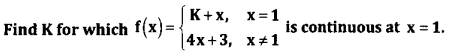$$\lim _{x \rightarrow 1} f(x)=f(1) \Rightarrow \lim _{x \rightarrow 1} 4 x+3=k+1$$
⇒ 4 + 3 = k + 1 ⇒ k = 6

Question 21.
Find $$\frac{\mathrm{d} y}{\mathrm{d} x}$$ if y = $$\sqrt{x+\sqrt{x+\sqrt{x+\dots \infty}}}$$
y = $$\sqrt{x+y}$$ y2 – 2y = x
$$\frac{d y}{d x}=\frac{1}{2 y-1}$$

Question 22.
If S = 5t2 +4t – 8.Find the initial velocity and acceleration.
v = 10t + 4 Initial velocity = 0 + 4 = 4
a = 10Question 23.
Evaluate: ∫(4x2 – 2x + 7)3/2 (4x – 1)dx.
Put 4x2 – 2x + 7 = t (8x – 2)dx = dt (4x-1)dx = $$\frac{\mathrm{dt}}{2}$$= $$\frac{1}{5}$$(4x2 – 2x + 7)5/2 + c

Question 24.
Evaluate: $$\int_{1}^{2} \frac{1}{x} d x$$
(log x)21 = log 2
Appendix B: Examination Question Papers L

Part – C

Answer any ten questions: (10 × 3 = 30 )

Question 25.
If A = $$\left[ \begin{matrix} -1 & 2 \\ 3 & 4 \end{matrix} \right]$$ show that A(adj A) = (adj A)A = |A|I.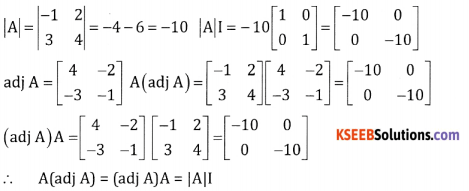Question 26.
Show that $$\left| \begin{matrix} -{ a }^{ 2 } & ab & bc \\ ab & -{ b }^{ 2 } & bc \\ ac & bc & -{ c }^{ 2 } \end{matrix} \right|$$ = 4a2b2c2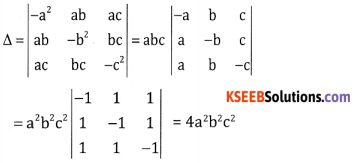Question 27.
A team of 11 is to be chosen from 18 cricketers of whom 6 are bowlers and 3 are wicket keepers. In how many ways can a team be chosen so that.
i. There are exactly 4 bowlers and one wicket keeper
ii. There are atleast 4 bowlers and atleast 2 wicket keepers.Question 28.
If A and B are event with P(A) = $$\frac { 5 }{ 8 }$$ P(B) = $$\frac { 3 }{ 8 }$$ and P(A ∪ B) = $$\frac { 3 }{ 4 }$$ Find
(1) P(B/A)
(ii) P(A/B)
P(A ∩ B) = P(A) + P(B) – P(A ∪ B)
$$=\frac{5}{8}+\frac{3}{8}-\frac{3}{4}=\frac{5+3-6}{8}=\frac{2}{8}$$Question 29.
Two taps can separately fill a tank in 12 min and 15 minutes respectively. The tank when full can be emptied by a drain pipe is 20 minutes. When the tank was empty, all the three were opened simultaneously. In what time will the tank be filled up?
1st tap can fill $$\frac{1}{12}$$th tank in 1 min
2nd tap can fill $$\frac{1}{15}$$th tank in 1 min
Drain pipe drain out $$\frac{1}{20}$$th tank in 1 min∴ the tank will get filled in 10 minutes.

Question 30.
The banker’s gain on a bill is $$\frac{1}{5}$$ of the banker’s discount and the rate of interest is 20% p.a. Find the unexpired period of the bill.
BG = $$\frac{1}{5}$$ BD r = 20% = 0.2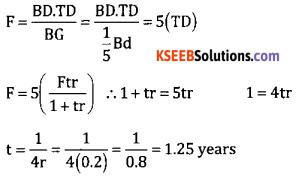Question 31.
Rakshith decides to invest in TCS shares which are selling at 2020 per share. How much money is required to purchase 10 shares, if the brokerage in 0.5%
Amount required to purchase 10 TCS shares = 2,020 × 10
= 20,200
Brokerage = 0.005 × 20,200 = 101
Net amount = 20,200 + 101 = 20,301

Question 32.
The price of a washing machine inclusive of sales tax is 13,530. If the sales tax is 10% . Find the basic price.
Suppose the basic price (MP) of the washing machine = Rs. x
∴ total amount paid = MP + ST % of MP
13,530 = x + 0.1x
x = 12,300

Question 33.
Find the length of the chord of the circle x2 + y2 – 6x – 4y – 12 = 0 on the coordinate axes.
Formulas
x = intercept = $$2 \sqrt{g^{2}-c}=2 \sqrt{9+12}=2 \sqrt{21}$$
y – intercept $$2 \sqrt{f^{2}-c}=2 \sqrt{4+12}=2 \sqrt{4+12}$$ = 8

Question 34.
If x = a sec θ, y = b tan θ find $$\frac{d y}{d x}$$ at θ = $$\frac{\pi}{4}$$
$$\frac{d y}{d x}=\frac{b \sec \theta}{a \tan \theta}$$Question 35.
The volume of a sphere is increasing at the rate 4π cc/sec, Find the rate at which the area of its surface increases when its radius is 10cm.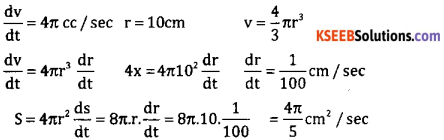Question 36.
Show that xx is minimum at x = $$\frac{1}{e}$$
y = xx $$\frac{\mathrm{d} y}{\mathrm{d} x}$$ = xx(1 +log x)
$$\frac{d^{2} y}{d x^{2}}=x^{x}\left(\frac{1}{x}\right)+x^{x}(1+\log x)^{2}=x^{x}\left\{\frac{1}{x}+(1+\log x)^{2}\right\}$$
For minima $$\frac{\mathrm{d} y}{\mathrm{d} x}$$ = 0 xx (1 + log x) = 0 ⇒ x = $$\frac{1}{e}$$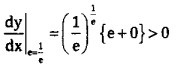∴ y is minimum at x = $$\frac{1}{e}$$Question 37.
Evaluate: ∫x2 log x dx.
∫x2 log xdx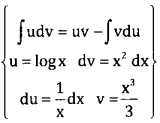$$=\frac{x^{2}}{3} \log x-\int \frac{x^{3}}{x} d x$$
$$=\frac{x^{3}}{3} \log x-\frac{x^{3}}{9}+c$$

Question 38.
Evaluate ∫cosec x[cosec x + cot x]dx.
∫(Cosec2x + Cosec xCotx)dx
= cot x – Cosec x + c

Part – D

Answer any six questions: (6 × 5 = 30 )

Question 39.
Evaluate (2 + √3)5 + (2 – 13)5
(2 + √3)5 + (2 – √3)5 = 2 [5C0 25 + 5C223 (√3)2 + 5C421 (√3)4 ]
= 2[32 + 240 + 90]
= 724

Question 40.
Resolve into partial fractions: $$\frac{3 x+5}{(x+2)(x-1)^{2}}$$3x + 5 = A(x – 1)2 + (x + 2)(x – 1) + C(x + 2)
A = $$\frac{-1}{9}$$ C = $$\frac{8}{3}$$ 0 = A + B B = $$\frac{1}{9}$$
Substitution of A,B,C

Question 41.
Verify whether the compound proposition p → p(~p ∨ q) is a tautology or a contradiction or neither.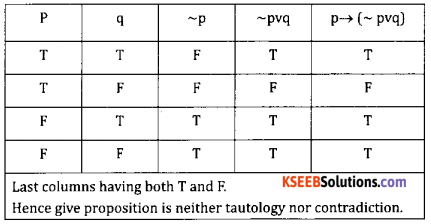Question 42.
If two men or four women can do a work in 33 days and 3 men and 5 women can do the same work in 24 days? How long shall 5 men and 2 women take to do the same work?
2 men and 4 women can do a work in 33 days.
66 men and 132 women can do a work in 1 day
Similarly 3 men and 5 women can do a work in 24 days
72 men and 120 women can do a work in 1 day
∴ 66 men + 132 women = 72 men + 120 women
∴ 1 man = 2 women
5 men + 2 women = 10 women + 2 women =12 women
Let the required number of days be x
2 men + 4 women = 4 women + 4 women = 8 women
8W : 12W = x : 33
12x = 33 × 8 ∴ x = 22 daysQuestion 43.
Sumsung company which manufacture LCD TV. The 1st lot of 10 units was completed in 1400 labour hours. Find each subsequent lot, the commutative production was doubled. And it has observed that 90% learning effect applies to all labour related cost. The anticipated production is 320 units of LCD TV find total labour cost required to manufacture 320 unit and also find the total labour cost at ₹ 20/hrs.
Let 10 units = 1 lot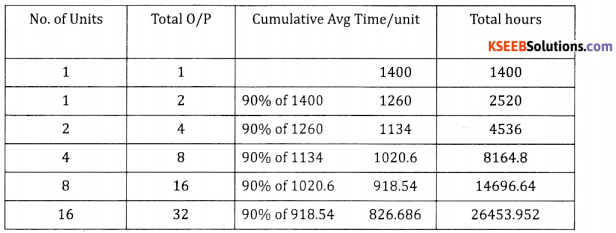Total labour hrs = 26453.952
Labour cost at Rs. 20/hr = 529079.04

Question 44.
Solve the LPP graphically:
Z = 3x + 5y
Subject to : x + 3y ≤ 3
x + y ≤ 2
x,y ≥ 0.
x + 3y = 3. A(3,0) B(0,1)
x + y =2 C(2,0) D(0,2)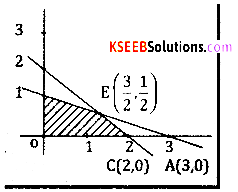Question 45.
In any triangle ABC, prove that
sin 2A + sin 2B – sin 2C = 4 cos A cos B sin C.
LHS = sin 2a + sin 2B – sin 2C
= 2sin (A + B) cos (A – B) – 2 sin C cos C
{A + B + C = 180° A + B = 180 – c}
{Sin(A + B) = Sinc Cos(A + B) = -Cosc}
LHS = 2 Sin C {Cos (A – B)-2 sin CCos C
= 2 sin C {Cos (A – B) + Cos(A + B)}
= 4 Cos A Cos B Sin C

Question 46.
Find the equation of the circle passing through the points (0,2) (3,0) and (3,2).
Let equation of circle be
x2 + y2 + 2gx + 2fy + c = 0 …. (1)
Here circle passing through (0,2)(3,0) and (3,2)
6g + C = -9 …. (2)
4f + C = 4 …. (3)
6g + 4f + c = -13 …. (4)
6g – 4 = -13 6g = -9 g = $$\frac{-3}{2}$$
4f – 9 = 13 4f = -4 f = -1
-4 + c = -4 c = 0
(1) ⇒ x2 + y2 – 6x – 2y = 0

Question 47.
If y – a cos (log x) + b sin (log x). Prove that x2y2 + xy1 + y = 0
y = a cos (log x) + b sin (log x)
y1 = $$\frac{-a \sin (\log x)}{x}+\frac{b \cos (\log x)}{x}$$
xy1 = – a sin (log x) +b cos(log x)
$$x y_{1}+y_{1}=\frac{-a \cos (\log x)}{x}+\frac{b \sin (\log x)}{x}$$
x2y2 + xy1 = – [a cos (log x) + b sin (log x)]
x2y2 + xy1 = -y
x2y2 + xy1 + y = 0Question 48.
Find the area enclosed between the curves y2 = x and x2 = y.
y2 = x … (1)
x2 = y … (2)Solving (1) and (2) we get
x=0 x = 1
Required area $$\int_{0}^{1}\left\{\sqrt{x}-x^{2}\right\} d x=\left\{\frac{2}{3} x^{\frac{3}{2}}-\frac{x^{3}}{3}\right\}_{0}^{1}$$
= $$\frac{2}{3}-\frac{1}{3}=\frac{1}{3}$$ sq.units

Part – E

Answer any six questions: (6 × 5 = 30 )

Question 49.
(a). A school wants to award its students for the values of punctuality, good behavior and hard work with a total cash award ₹ 6,000. Three time the award money for hard work together with the award money for punctuality is ₹ 11,000 the award money for punctuality and hard work together is double the one given for Good Behavior. Represent the above situation algebraically and also find the award money
for each value. Using matrix method.
Let the values of punctuality. Good Behavior and Hard work be denote by x,y and z respectively
x + y + z = 6000
x + 32 = 11000
x – 2y + z = 0
Its matrix form is Ax = B where∴ x = ₹ 500 y = ₹ 2000 ∴ z = ₹3500

b. Find the value of (1,01)5 using Bonomial upto 4 decimal places.
(1.01)5 = (1 + 0.01)5
= 1 + 5C1(0.01) + 5C2(0.01)2 + 5C3 (0.01)3 + 5C4 (0.01)4 + 5C5 (0.01)5
= 1 + 5(0.01)+10(0.001)+10(0.000001) + ignoring the further terms
= 1.0510[correct upto 4 decimals]

Question 50.
(a). If n is a rational number and ‘a’ is non-zero real number, then prove that $$\lim _{x \rightarrow a} \frac{x^{n}-a^{n}}{x-a}=n a^{n-1}$$

Case – 1 : Let n be a positive integer.
xn – an = (x – a)(xn -1 + xn-2 . a + xn-3 . a2 + ……. + an-1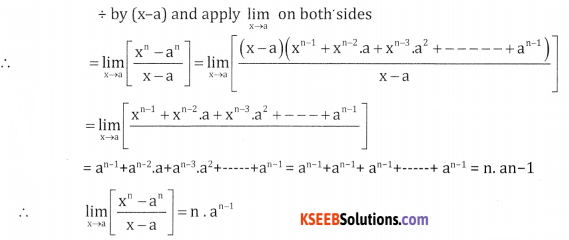Case – 2: Let n be a positive integer
Put n = -m, m > 0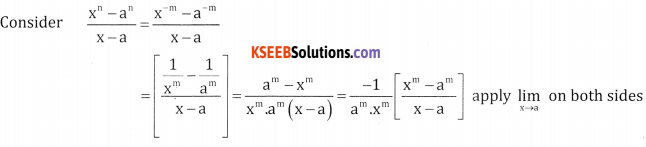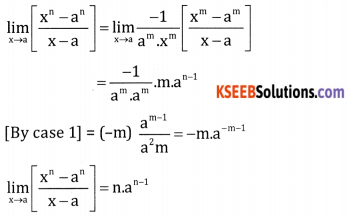Case – 3: Let n = p/q where p and q are integers and q ≠ 0(b). The angles of depression of two boats as observed from the mast head of a ship 50m high are 45o and 30°. What is the distance between the boats if they are on the same side of the mast head in line with it?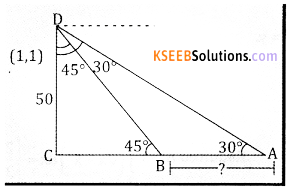From triangle BCD, tan 45° = $$\frac{50}{\mathrm{CB}}$$ ∴ CB = 50
From triangle ACD, tan 30°= $$\frac{50}{\mathrm{AC}}$$ AC = 50√3